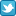Search DSS

Finding Data Analyzing Data Citing data

DSS lab consultation schedule
(Monday-Friday)
 Sep 1-Oct 16 By Zoom appt. here Oct 19-Dec 4 Virtual Zoom Walk-ins Dec 7-Jan 31 By Zoom appt. here Feb 1-April 30 Virtual Zoom Walk-ins May 3-Aug 31 By appt. here
For quick questions email data@princeton.edu.
*No appts. necessary during walk-in hrs.
Note: the DSS lab is open as long as Firestone is open, no appointments necessary to use the lab computers for your own analysis.HomeOnline helpStatistical PackagesS-PlusAnalysis

# Basic Analysis in S-Plus

Here's our sample data file again, in comma-delimited format. Copy it to a text file, save it and read it into an S-Plus object called data to follow along with the tutorial.

```country,ud,sd
Sweden,82.4,111.84
Israel,80,73.17
Iceland,74.3,17.25
Finland,73.3,59.33
Belgium,71.9,43.25
Denamrk,69.8,90.24
Ireland,68.1,0
Austria,65.6,48.67
NZ,59.4,60
Norway,58.9,83.08
Australia,51.4,33.74
Italy,50.6,0
UK,48,43.67
Germany,39.6,35.33
Netherlands,37.7,31.50
Switzerland,35.4,11.87
Japan,31,1.92
France,28.2,8.67
USA,24.5,0```

## Referring to Variables

Apart from the identifier country, there are two variables in this data set: union density (ud) and social democratic government ( sd). S-Plus gives you several ways to refer to variables in a data.frame. S-Plus recognizes a \$ as indicating a sub-object for a particular object. For example, if you type data\$ud you will get a listing of the union density variable.

A simple way to make the variables available is to attach the data frame, which makes the variables available act as if they were objects themselves:

attach(data)

Now the variables can be referred to simply by their names.

## Summary Statistics

You might want to know some things about these variables, like their mean, range, standard deviation, and so forth. Several commands provide convenient ways to extract basic summary statistics. They are: mean(), median(), cor(), var(), and summary(). The command summary(object) is the most useful because it outputs several statistics of interest.

Now let's move on to regression.

## Regression - Linear Models using lm()

The lm() function of S-Plus fits a simple linear regression model based on several parameters. Here is a description of a basic command, and a listing of those parameters:

out1 <- lm(dependentvar ~ independvar1 + independvar2 + ...,
data = dataframe,
na.action = na.fail)

Note that all S-Plus commands which fit a model include a ~ in the equation. The ~ separates the dependent variable from the independent variables. Now

variables
dependent and independent variables need to be specified.
data =
Specifies the data frame in which the variables reside. Again, this is not necessary if the data elements are independent objects in your directory, or if you have used the attach() command to attach your data frame.
na.action =
This tells Splus how to deal with missing values. The default value is na.fail which means that the command will fail if there is missing data. If you want it to eliminate the observations for which there is missing data, you have to type na.action=na.omit.
other commands
Type ?lm to get help and see all the possible subcommands for lm.

Lets do an example with the data from before so that we can understand the different parts of regression output.

out1 <- lm(ud ~ sd, data = data)

Learning to read the output and get the full extent of output from Splus is very important. To see the model you just created, you can type out1 and it will give you some output which is not particularly interesting. It does not give us significance tests and other useful information. We can get this information with the summary() command. To see the object we just created type summary(out1). You should see the following output.

```Call: lm(formula = ud ~ sd, data = data1)
Residuals:
Min     1Q Median    3Q   Max
-15.38 -10.27 -3.558 10.81 28.22

Coefficients:
Value Std. Error t value Pr(>|t|)
(Intercept) 39.8841  4.8127     8.2873  0.0000
sd  0.3764  0.0962     3.9131  0.0010

Residual standard error: 14.16 on 18 degrees of freedom
Multiple R-Squared: 0.4597
F-statistic: 15.31 on 1 and 18 degrees of freedom, the p-value is 0.001019

Correlation of Coefficients:
(Intercept)
sd -0.753
```

Notice all the pieces of information. First it gives you the call or formula and specifications that S-Plus uses to create the linear model object. Next it gives you summary statistics of the residuals which we will not deal with for now. Then note that it gives you coefficients, standard errors, and t-values and their associated probabilities for each of the variables in your equation. These you should know how to read. Remember that in general you're looking for significance levels less than .05. Then you get some statistics for the model: residual standard error, multiple R-squared, and the F-statistics with its associated probability. Finally you're given the correlation of the coefficients in your model.

There are some good things to know about the way Splus works. Do you remember that you can extract sub-objects with a \$. We used this to look at individual variables in a data frame. We can also use it to look at sub-objects of regression output. Before we do that, we need to know what all the sub-objects are. To find this out, type names(out1) You should see a list of subobjects like this:

```  "coefficients"  "residuals"     "fitted.values" "effects"
 "R"             "rank"          "assign"        "df.residual"
 "contrasts"     "terms"         "call"
```

Each of these subobjects can now be extracted. For example, if we are interested in the residual values, we can type out1\$residuals to see the residuals. This will be very convenient when you need to do diagnostics.